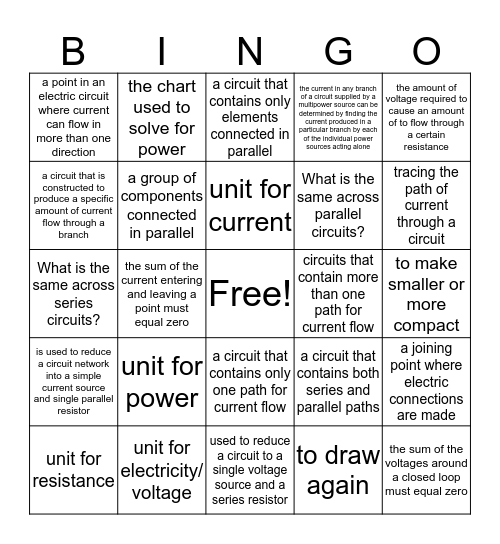# Unit 8 & 9 VocabularyThis bingo card has a free space and 24 words: unit for power, unit for electricity/ voltage, unit for current, unit for resistance, the amount of voltage required to cause an amount of to flow through a certain resistance, a point in an electric circuit where current can flow in more than one direction, a circuit that is constructed to produce a specific amount of current flow through a branch, the chart used to solve for power, circuits that contain more than one path for current flow, a circuit that contains only one path for current flow, What is the same across parallel circuits?, What is the same across series circuits?, a circuit that contains both series and parallel paths, a joining point where electric connections are made, a group of components connected in parallel, to draw again, to make smaller or more compact, a circuit that contains only elements connected in parallel, tracing the path of current through a circuit, the sum of the current entering and leaving a point must equal zero, the sum of the voltages around a closed loop must equal zero, is used to reduce a circuit network into a simple current source and single parallel resistor, the current in any branch of a circuit supplied by a multipower source can be determined by finding the current produced in a particular branch by each of the individual power sources acting alone and used to reduce a circuit to a single voltage source and a series resistor.

## Play Online